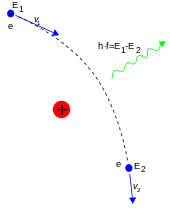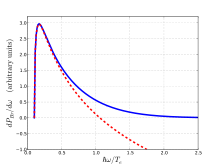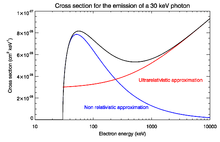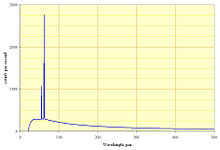# Bremsstrahlung

﻿
BremsstrahlungBremsstrahlung produced by a high-energy electron deflected in the electric field of an atomic nucleus

Bremsstrahlung (German pronunciation: [ˈbʁɛmsˌʃtʁaːlʊŋ] , from bremsen "to brake" and Strahlung "radiation", i.e. "braking radiation" or "deceleration radiation") is electromagnetic radiation produced by the deceleration of a charged particle when deflected by another charged particle, typically an electron by an atomic nucleus. The moving particle loses kinetic energy, which is converted into a photon because energy is conserved. The term is also used to refer to the process of producing the radiation. Bremsstrahlung has a continuous spectrum, which becomes more intense and shifts toward higher frequencies when the energy of the accelerated particles is increased.

Strictly speaking, bremsstrahlung refers to any radiation due to the acceleration of a charged particle, which includes synchrotron radiation; however, it is frequently used in the more narrow sense of radiation from electrons stopping in matter.

Bremsstrahlung emitted from plasma is sometimes referred to as free-free radiation. This refers to the fact that the radiation in this case is created by charged particles that are free both before and after the deflection (acceleration) that causes the emission.

## Dipole approximation

Suppose that a particle of charge q experiences an acceleration$\vec{a}$ which is collinear with its velocity$\vec{v}$ (this is the relevant case for linear accelerators). Then, the relativistic expression for the angular distribution of the bremsstrahlung (considering only the dominant dipole radiation contribution), is$\frac{dP(\theta)}{d\Omega} = \frac{q^2 a^2}{16 \pi^2 \epsilon_0 c^3}\frac{{\sin^2{\theta}}}{(1 - \beta \cos{\theta})^5}$,
where β = v / c and θ is the angle between$\vec{a}$ and the point of observation.

Integrating over all angles then gives the total power emitted as$P_{a \parallel v} = \frac{q^2 a^2 \gamma^6}{6 \pi \epsilon_0 c^3}$,

where γ is the Lorentz factor .

The general expression for the total radiated power is$P = \frac{q^2 \gamma^4}{6 \pi \epsilon_0 c} \left( \dot{\beta}^2 + \frac{(\vec{\beta} \cdot \dot{\vec{\beta}})^2}{1 - \beta^2}\right)$

where$\dot{\beta}$ signifies a time derivative of β. Note, this general expression for total radiated power simplifies to the above expression for the specific case of acceleration parallel to velocity ($\vec{\beta} \cdot \dot{\vec{\beta}} = \beta \dot{\beta}$), by noting that$\dot{\beta} = a/c$ and γ = (1 − β2) − 1 / 2. For the case of acceleration perpendicular to the velocity ($\vec{\beta} \cdot \dot{\vec{\beta}} = 0$) (a case that arises in circular particle accelerators known as synchrotrons), the total power radiated reduces to$P_{a \perp v} = \frac{q^2 a^2 \gamma^4 }{6 \pi \epsilon_0 c^3}$.

The total power radiated in the two limiting cases is proportional to γ4 ($a \perp v$) or γ6 ($a \parallel v$). Since E = γmc2, we see that the total radiated power goes as m − 4 or m − 6, which accounts for why electrons lose energy to bremsstrahlung radiation much more rapidly than heavier charged particles (e.g., muons, protons, alpha particles). This is the reason a TeV energy electron-positron collider (such as the proposed International Linear Collider) cannot use a circular tunnel (requiring constant acceleration), while a proton-proton collider (such as the Large Hadron Collider) can utilize a circular tunnel. The electrons lose energy due to bremsstrahlung at a rate$(m_p/m_e)^4 \approx 10^{13}$ times higher than protons do.

## Thermal bremsstrahlungThe bremsstrahlung power spectrum rapidly decreases for large ω, and is also suppressed near ω = ωp. This plot is for the quantum case Te > Z2Eh, and$\hbar\omega_p/T_e=0.1$.

In a plasma the free electrons constantly produce bremsstrahlung in collisions with the ions. A complete analysis requires accounting for both binary Coulomb collisions as well as collective (dielectric) behavior. A detailed treatment is given in, some of which is summarized in [?], while a simplified one is given in. In this section we follow Bekefi's dielectric treatment, with collisions included approximately via the cutoff wavenumber km.

Consider a uniform plasma, with thermal electrons (distributed according to the Maxwell–Boltzmann distribution with the temperature Te). Following Bekefi, the power spectral density (power per angular frequency interval per volume, integrated over the whole sr of solid angle, and in both polarizations) of the bremsstrahlung radiated, is calculated to be${dP_\mathrm{Br} \over d\omega} = {8\sqrt 2 \over 3\sqrt\pi} \left[1-{\omega_p^2 \over \omega^2}\right]^{1/2} \left[ Z_i^2 n_i n_e r_e^3 \right] \left[ { \frac{(m_ec^2)^{3/2}}{(k_B T_e)^{1/2}} } \right] E_1(y) ,$

where ωp is the electron plasma frequency, ne,ni is the number density of electrons and ions, re is the classical radius of electron, me is its mass, kB is the Boltzmann constant, and c is the speed of light. The first bracketed factor is the index of refraction of a light wave in a plasma, and shows that emission is greatly suppressed for ω < ωp (this is the cutoff condition for a light wave in a plasma; in this case the light wave is evanescent). This formula thus only applies for ω > ωp. Note that the second bracketed factor has units of 1/volume and the third factor has units of energy, giving the correct total units of energy/volume. This formula should be summed over ion species in a multi-species plasma.

The special function E1 is defined in the exponential integral article, and the unitless quantity y is$y = {1\over 2}{\omega^2 m_e \over k_m^2 k_B T_e}$

km is a maximum or cutoff wavenumber, arising due to binary collisions, and can vary with ion species. Roughly, km = 1 / λB when$k_B T_e>Z_i^2 E_h$ (typical in plasmas that are not too cold), where$E_h \approx 27.2$ eV is the Hartree energy, and$\lambda_B=\hbar/(m_e k_B T_e)^{1/2}$ is the electron thermal de Broglie wavelength. Otherwise,$k_m \propto 1/l_c$ where lc is the classical Coulomb distance of closest approach.

For the usual case km = 1 / λB, we find$y = {1\over2}\left[\frac{\hbar\omega}{k_B T_e}\right]^2$ .

The formula for dPBr / dω is approximate, in that it neglects enhanced emission occurring for ω slightly above ωp.

In the limit y < < 1, we can approximate E1 as$E_1(y) \approx -\ln [y e^\gamma] + O(y)$ where$\gamma\approx 0.577$ is the Euler-Mascheroni constant. The leading, logarithmic term is frequently used, and resembles the Coulomb logarithm that occurs in other collisional plasma calculations. For y > e − γ the log term is negative, and the approximation is clearly inadequate. Bekefi gives corrected expressions for the logarthmic term that match detailed binary-collision calculations.

The total emission power density, integrated over all frequencies, is\begin{align} P_\mathrm{Br} &= \int_{\omega_p}^\infty d\omega {dP_\mathrm{Br}\over d\omega} = {16 \over 3} \left[ Z_i^2 n_i n_e r_e^3 \right] \left[m_e c^3 \right] k_m G(y_p) \\ G(y_p) &= {1 \over 2\sqrt{\pi}} \int_{y_p}^\infty dy y^{-1/2} \left[1-{y_p\over y}\right]^{1/2} E_1(y) \\ y_p &= y(\omega=\omega_p) \end{align}

G(yp = 0) = 1 and decreases with yp; it is always positive. For km = 1 / λB, we find$P_\mathrm{Br} = {16 \over 3} \left[ Z_i^2 n_i n_e r_e^3 \right] \left[ {c \over r_e} (m_e c^2 k_B T_e)^{1/2} \right] \alpha G(y_p)$

The first bracketed factor has units of 1/volume, while the second has units of power. Note the appearance of the fine structure constant α due to the quantum nature of λB. In practical units, a commonly used version of this formula for G = 1 is$P_\mathrm{Br} [\textrm{W/m}^3] = {Z_i^2 n_i n_e \over \left[7.69 \times 10^{18} \textrm{m}^{-3}\right]^2} T_e[\textrm{eV}]^{1/2}$ .

This formula is 1.59 times the one given above, with the difference due to details of binary collisions. Such ambiguity is often expressed by introducing Gaunt factor gB, e.g. in  one finds$\epsilon_\mathrm{ff} = 1.4\times 10^{-27} T^{1/2} n_{e} n_{i} Z^{2} g_B\,,$

where everything is expressed in the CGS units.

### Relativistic correctionsRelativistic corrections to the emission of a 30-keV photon by an electron impacting on a proton.

For very high temperatures there are relativistic corrections to this formula, that is, additional terms of the order of$k_B T_e/m_e c^2\,.$

### Bremsstrahlung cooling

If the plasma is optically thin, the bremsstrahlung radiation leaves the plasma, carrying part of the internal plasma energy. This effect is known as the bremsstrahlung cooling. It is a type of radiative cooling. The energy carried away by bremsstrahlung is called bremsstrahlung losses and represent, respectively, a type of radiative losses. One generally uses the term bremsstrahlung losses in the context when the plasma cooling is undesired, as e.g. in fusion plasmas.

## Sources of bremsstrahlung

### X-ray tubeSpectrum of the X-rays emitted by an X-ray tube with a rhodium target, operated at 60 kV. The continuous curve is due to bremsstrahlung, and the spikes are characteristic K lines for rhodium. The curve goes to zero at 21 pm in agreement with the Duane–Hunt law, as described in the text.

In an X-ray tube, electrons are accelerated in a vacuum by an electric field and shot into a piece of metal called the "target". X-rays are emitted as the electrons slow down (decelerate) in the metal. The output spectrum consists of a continuous spectrum of X-rays, with additional sharp peaks at certain energies (see graph on right). The continuous spectrum is due to bremsstrahlung, while the sharp peaks are characteristic X-rays associated with the atoms in the target. For this reason, bremsstrahlung in this context is also called continuous X-rays.

The spectrum has a sharp cutoff at low wavelength, which is due to the limited energy of the incoming electrons. For example, if an electron in the tube is accelerated through 60 kV, then it will acquire a kinetic energy of 60 keV, and when it strikes the target it can create X-rays with energy of at most 60 keV, by conservation of energy. (This upper limit corresponds to the electron coming to a stop by emitting just one X-ray photon. Usually the electron emits many photons, and each has an energy less than 60 keV.) A photon with energy of at most 60 keV has wavelength of at least 21 pm, so the continuous X-ray spectrum has exactly that cutoff, as seen in the graph. More generally the formula for the low-wavelength cutoff is:$\lambda_{\text{min}} = \frac{h c}{e V} \approx \frac{1239.8 \text{ pm}}{V\text{ in kV}} \,$

where h is Planck constant, c is the speed of light, V is the voltage that the electrons are accelerated through, e is the elementary charge, and pm is picometers. This is called the Duane–Hunt law.

### Beta decay

Beta particle-emitting substances sometimes exhibit a weak radiation with continuous spectrum that is due to bremsstrahlung. In this context, bremsstrahlung is a type of "secondary radiation", in that it is produced as a result of stopping (or slowing) the primary radiation (beta particles). In electron and positron emission the photon's energy comes from the electron/nucleon pair, with the spectrum of the bremsstrahlung decreasing continuously with increasing energy of the beta particle. In electron capture the energy comes at the expense of the neutrino, and the spectrum is greatest at about one third of the normal neutrino energy, reaching zero at zero energy and at normal neutrino energy.

#### Inner and outer bremsstrahlung

The "inner" bremsstrahlung arises from the creation of the electron and its loss of energy (due to the strong electric field in the region of the nucleus undergoing decay) as it leaves the nucleus. This is to be contrasted with the "outer" bremsstrahlung due to the impingement on the nucleus of electrons coming from the outside (i.e., emitted by another nucleus).

In some cases, e.g. 32P, the bremsstrahlung produced by shielding the beta radiation with the normally used dense materials (e.g. lead) is itself dangerous; in such cases, shielding must be accomplished with low density materials, e.g. Plexiglass (lucite), plastic, wood, or water; because the rate of deceleration of the electron is slower, the radiation given off has a longer wavelength and is therefore less penetrating.

### In astrophysics

The dominant luminous component in a cluster of galaxies is the 107 to 108 kelvin intracluster medium. The emission from the intracluster medium is characterized by thermal bremsstrahlung. This radiation is in the energy range of X-rays and can be easily observed with space-based telescopes such as Chandra X-ray Observatory, XMM-Newton, ROSAT, ASCA, EXOSAT, Suzaku, RHESSI and future missions like IXO  and Astro-H .

Bremsstrahlung is also the dominant emission mechanism for HII Regions at radio wavelengths.

Wikimedia Foundation. 2010.

### Look at other dictionaries:

• Bremsstrahlung — Saltar a navegación, búsqueda Bremsstrahlung (del alemán bremsen frenar y Strahlung radiación , o sea, radiación de frenado ) es una radiación electromagnética producida por la deceleración de una partícula cargada, como por ejemplo un electrón,… …   Wikipedia Español

• bremsstrahlung — ● bremsstrahlung nom féminin (mot allemand, de Bremsen, freinage, et Strahlung, rayonnement) Synonyme de rayonnement de freinage. ● bremsstrahlung (synonymes) nom féminin (mot allemand, de Bremsen, freinage, et Strahlung, rayonnement) Synonymes …   Encyclopédie Universelle

• bremsstrahlung — [brem′shträ΄looŋ] n. [Ger, lit., braked radiation < bremse, a brake + strahlung, radiation] the electromagnetic radiation given off by a high energy particle, as an electron, when suddenly accelerated or retarded by an electric field or by… …   English World dictionary

• Bremsstrahlung — Erzeugung von Röntgenbremsstrahlung durch Abbremsung eines schnellen Elektrons in dem Coulombfeld eines Atomkerns (schematische Darstellung) Bremsstrahlung ist die elektromagnetische Strahlung, die entsteht, wenn ein geladenes Teilchen, zum… …   Deutsch Wikipedia

• Bremsstrahlung — Brẹms|strah|lung 〈f. 20; Phys.〉 die beim Auftreffen geladener Elementarteilchen auf Materie entstehende elektromagnetische Strahlung, z. B. Röntgen , Gammastrahlung; Ggs Eigenstrahlung * * * Brẹms|strah|lung: beim Beschleunigen oder (häufiger)… …   Universal-Lexikon

• Bremsstrahlung — Rayonnement continu de freinage Le rayonnement continu de freinage ou Bremsstrahlung (aussi appelé free free emission en anglais) est un rayonnement électromagnétique à spectre large créé par le ralentissement de charges électriques. On parle… …   Wikipédia en Français

• bremsstrahlung — /brem shtrah leuhng/, n. Physics. radiation emitted by a charged particle when accelerating, as x rays emitted by an electron that is scattered by a nucleus. [1940 45; < G, equiv. to Brems(e) brake + Strahlung radiation] * * * (German; braking… …   Universalium

• Bremsstrahlung — stabdomasis rentgeno spinduliavimas statusas T sritis fizika atitikmenys: angl. braking X radiation; bremsstrahlung vok. Bremsröntgenstrahlung, f; Bremsstrahlung, f; Röntgenbremsstrahlung, f rus. рентгеновское тормозное излучение, n; тормозное… …   Fizikos terminų žodynas

• bremsstrahlung — stabdomasis rentgeno spinduliavimas statusas T sritis fizika atitikmenys: angl. braking X radiation; bremsstrahlung vok. Bremsröntgenstrahlung, f; Bremsstrahlung, f; Röntgenbremsstrahlung, f rus. рентгеновское тормозное излучение, n; тормозное… …   Fizikos terminų žodynas

• bremsstrahlung — /ˈbrɛmstralʊŋ/ (say bremstrahloong) noun 1. X ray radiation produced from the rapid deceleration of electrons as they enter a heavy metal target. 2. any radiation produced by change in electron velocity. Also, bremsstrahlung radiation. {German:… …   Australian English dictionary